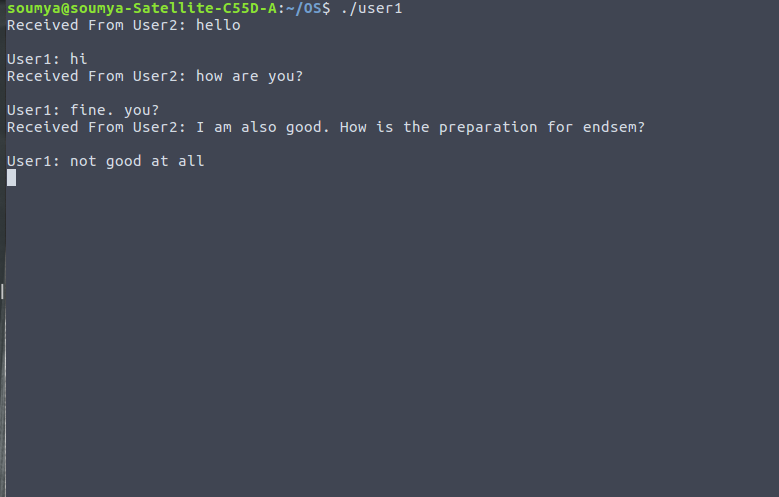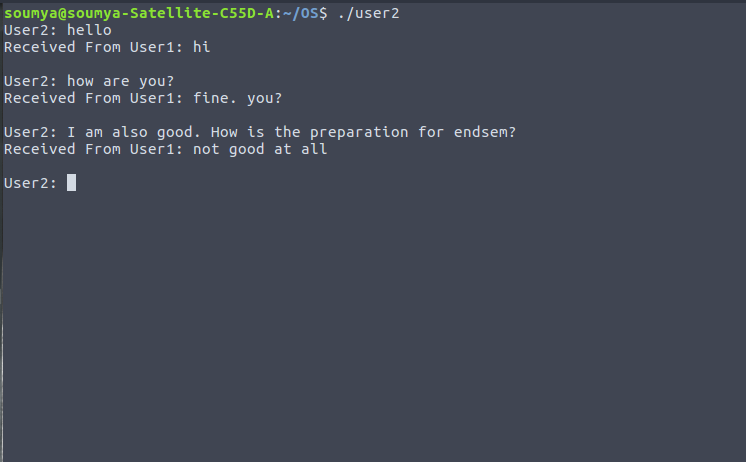GFG App
Open AppBrowser
Continue

# Chat application between two processes using signals and shared memory

Prerequisite: C signal handling, IPC through shared memory

A signal is used in the UNIX system to notify a process that a particular event has occurred. A signal may be received either synchronously or asynchronously depending on the source and the reason for the event being signalled. A signal must follow the following pattern –

1. A signal is generated by the occurrence of a particular event.

2. A generated signal is delivered to a particular process.

3. The signal must be handled after receiving at the process.

In this problem, the message is sent from one user to another user using kill function. kill function takes two inputs – process id of the receiver process and signal type. For this purpose, we use a shared memory where we store the process id(s) of two processes. We use a handler function which will print the message received from another process. User2 will start to send message to User1 and then they will continue chatting.

## User 1

 `#include ` `#include ` `#include ` `#include ` `#include ` `#include ` `#include ` `#include ` ` `  `#define FILLED 0 ` `#define Ready 1 ` `#define NotReady -1 ` ` `  `struct` `memory { ` `    ``char` `buff; ` `    ``int` `status, pid1, pid2; ` `}; ` ` `  `struct` `memory* shmptr; ` ` `  `// handler function to print message received from user2 ` ` `  `void` `handler(``int` `signum) ` `{ ` `    ``// if signum is SIGUSR1, then user 1 is receiving a message from user2 ` ` `  `    ``if` `(signum == SIGUSR1) { ` `        ``printf``(``"Received User2: "``); ` `        ``puts``(shmptr->buff); ` `    ``} ` `} ` ` `  `int` `main() ` `{ ` `    ``// process id of user1 ` ` `  `    ``int` `pid = getpid(); ` ` `  `    ``int` `shmid; ` ` `  `    ``// key value of shared memory ` `    ``int` `key = 12345; ` ` `  `    ``// shared memory create ` `    ``shmid = shmget(key, ``sizeof``(``struct` `memory), IPC_CREAT | 0666); ` ` `  `    ``// attaching the shared memory ` ` `  `    ``shmptr = (``struct` `memory*)shmat(shmid, NULL, 0); ` ` `  `    ``// store the process id of user1 in shared memory ` `    ``shmptr->pid1 = pid; ` `    ``shmptr->status = NotReady; ` ` `  `    ``// calling the signal function using signal type SIGUSER1 ` `    ``signal``(SIGUSR1, handler); ` ` `  `    ``while` `(1) { ` `        ``while` `(shmptr->status != Ready) ` `            ``continue``; ` `        ``sleep(1); ` ` `  `        ``// taking input from user1 ` ` `  `        ``printf``(``"User1: "``); ` `        ``fgets``(shmptr->buff, 100, stdin); ` ` `  `        ``shmptr->status = FILLED; ` ` `  `        ``// sending the message to user2 using kill function ` ` `  `        ``kill(shmptr->pid2, SIGUSR2); ` `    ``} ` ` `  `    ``shmdt((``void``*)shmptr); ` `    ``shmctl(shmid, IPC_RMID, NULL); ` `    ``return` `0; ` `} `

## User 2

 `#include ` `#include ` `#include ` `#include ` `#include ` `#include ` `#include ` `#include ` ` `  `#define FILLED 0 ` `#define Ready 1 ` `#define NotReady -1 ` ` `  `struct` `memory { ` `    ``char` `buff; ` `    ``int` `status, pid1, pid2; ` `}; ` ` `  `struct` `memory* shmptr; ` ` `  `// handler function to print message received from user1 ` ` `  `void` `handler(``int` `signum) ` `{ ` `    ``// if signum is SIGUSR2, then user 2 is receiving a message from user1 ` ` `  `    ``if` `(signum == SIGUSR2) { ` `        ``printf``(``"Received From User1: "``); ` `        ``puts``(shmptr->buff); ` `    ``} ` `} ` ` `  `// main function ` ` `  `int` `main() ` `{ ` `    ``// process id of user2 ` `    ``int` `pid = getpid(); ` ` `  `    ``int` `shmid; ` ` `  `    ``// key value of shared memory ` `    ``int` `key = 12345; ` ` `  `    ``// shared memory create ` ` `  `    ``shmid = shmget(key, ``sizeof``(``struct` `memory), IPC_CREAT | 0666); ` ` `  `    ``// attaching the shared memory ` ` `  `    ``shmptr = (``struct` `memory*)shmat(shmid, NULL, 0); ` ` `  `    ``// store the process id of user2 in shared memory ` `    ``shmptr->pid2 = pid; ` ` `  `    ``shmptr->status = NotReady; ` ` `  `    ``// calling the signal function using signal type SIGUSR2 ` `    ``signal``(SIGUSR2, handler); ` ` `  `    ``while` `(1) { ` `        ``sleep(1); ` ` `  `        ``// taking input from user2 ` ` `  `        ``printf``(``"User2: "``); ` `        ``fgets``(shmptr->buff, 100, stdin); ` `        ``shmptr->status = Ready; ` ` `  `        ``// sending the message to user1 using kill function ` ` `  `        ``kill(shmptr->pid1, SIGUSR1); ` ` `  `        ``while` `(shmptr->status == Ready) ` `            ``continue``; ` `    ``} ` ` `  `    ``shmdt((``void``*)shmptr); ` `    ``return` `0; ` `} `

Output:

••My Personal Notes arrow_drop_up